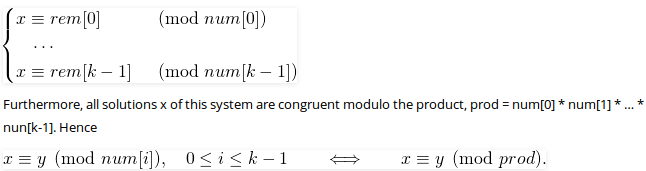# Chinese Remainder Theorem | Set 1 (Introduction)

• Difficulty Level : Easy
• Last Updated : 23 Aug, 2021

We are given two arrays num[0..k-1] and rem[0..k-1]. In num[0..k-1], every pair is coprime (gcd for every pair is 1). We need to find minimum positive number x such that:

```     x % num    =  rem,
x % num    =  rem,
.......................
x % num[k-1]  =  rem[k-1] ```

Basically, we are given k numbers which are pairwise coprime, and given remainders of these numbers when an unknown number x is divided by them. We need to find the minimum possible value of x that produces given remainders.
Examples :

```Input:  num[] = {5, 7}, rem[] = {1, 3}
Output: 31
Explanation:
31 is the smallest number such that:
(1) When we divide it by 5, we get remainder 1.
(2) When we divide it by 7, we get remainder 3.

Input:  num[] = {3, 4, 5}, rem[] = {2, 3, 1}
Output: 11
Explanation:
11 is the smallest number such that:
(1) When we divide it by 3, we get remainder 2.
(2) When we divide it by 4, we get remainder 3.
(3) When we divide it by 5, we get remainder 1.```

Chinese Remainder Theorem states that there always exists an x that satisfies given congruences. Below is theorem statement adapted from wikipedia
Let num, num, …num[k-1] be positive integers that are pairwise coprime. Then, for any given sequence of integers rem, rem, … rem[k-1], there exists an integer x solving the following system of simultaneous congruences.The first part is clear that there exists an x. The second part basically states that all solutions (including the minimum one) produce the same remainder when divided by-product of n, num, .. num[k-1]. In the above example, the product is 3*4*5 = 60. And 11 is one solution, other solutions are 71, 131, .. etc. All these solutions produce the same remainder when divided by 60, i.e., they are of form 11 + m*60 where m >= 0.
A Naive Approach to find x is to start with 1 and one by one increment it and check if dividing it with given elements in num[] produces corresponding remainders in rem[]. Once we find such an x, we return it.
Below is the implementation of Naive Approach.

## C++

 `// A C++ program to demonstrate working of Chinise remainder``// Theorem``#include``using` `namespace` `std;` `// k is size of num[] and rem[].  Returns the smallest``// number x such that:``//  x % num = rem,``//  x % num = rem,``//  ..................``//  x % num[k-2] = rem[k-1]``// Assumption: Numbers in num[] are pairwise coprime``// (gcd for every pair is 1)``int` `findMinX(``int` `num[], ``int` `rem[], ``int` `k)``{``    ``int` `x = 1; ``// Initialize result` `    ``// As per the Chinese remainder theorem,``    ``// this loop will always break.``    ``while` `(``true``)``    ``{``        ``// Check if remainder of x % num[j] is``        ``// rem[j] or not (for all j from 0 to k-1)``        ``int` `j;``        ``for` `(j=0; j

## Java

 `// A Java program to demonstrate the working of Chinese remainder``// Theorem``import` `java.io.*;` `class` `GFG {``    ` `    ``// k is size of num[] and rem[].  Returns the smallest``    ``// number x such that:``    ``//  x % num = rem,``    ``//  x % num = rem,``    ``//  ..................``    ``//  x % num[k-2] = rem[k-1]``    ``// Assumption: Numbers in num[] are pairwise coprime``    ``// (gcd for every pair is 1)``    ``static` `int` `findMinX(``int` `num[], ``int` `rem[], ``int` `k)``    ``{``        ``int` `x = ``1``; ``// Initialize result``     ` `        ``// As per the Chinese remainder theorem,``        ``// this loop will always break.``        ``while` `(``true``)``        ``{``            ``// Check if remainder of x % num[j] is``            ``// rem[j] or not (for all j from 0 to k-1)``            ``int` `j;``            ``for` `(j=``0``; j

## Python3

 `# A Python3 program to demonstrate``# working of Chinise remainder Theorem` `# k is size of num[] and rem[].``# Returns the smallest number x``# such that:``# x % num = rem,``# x % num = rem,``# ..................``# x % num[k-2] = rem[k-1]``# Assumption: Numbers in num[]``# are pairwise coprime (gcd for``# every pair is 1)``def` `findMinX(num, rem, k):``    ``x ``=` `1``; ``# Initialize result` `    ``# As per the Chinise remainder``    ``# theorem, this loop will``    ``# always break.``    ``while``(``True``):``        ` `        ``# Check if remainder of``        ``# x % num[j] is rem[j]``        ``# or not (for all j from``        ``# 0 to k-1)``        ``j ``=` `0``;``        ``while``(j < k):``            ``if` `(x ``%` `num[j] !``=` `rem[j]):``                ``break``;``            ``j ``+``=` `1``;` `        ``# If all remainders``        ``# matched, we found x``        ``if` `(j ``=``=` `k):``            ``return` `x;` `        ``# Else try next number``        ``x ``+``=` `1``;` `# Driver Code``num ``=` `[``3``, ``4``, ``5``];``rem ``=` `[``2``, ``3``, ``1``];``k ``=` `len``(num);``print``(``"x is"``, findMinX(num, rem, k));` `# This code is contributed by mits`

## C#

 `// C# program to demonstrate working``// of Chinise remainder Theorem``using` `System;` `class` `GFG``{``    ` `    ``// k is size of num[] and rem[].``    ``// Returns the smallest``    ``// number x such that:``    ``// x % num = rem,``    ``// x % num = rem,``    ``// ..................``    ``// x % num[k-2] = rem[k-1]``    ``// Assumption: Numbers in num[]``    ``// are pairwise coprime``    ``// (gcd for every pair is 1)``    ``static` `int` `findMinX(``int` `[]num, ``int` `[]rem,``                        ``int` `k)``    ``{``        ` `        ``// Initialize result``        ``int` `x = 1;``    ` `        ``// As per the Chinese remainder theorem,``        ``// this loop will always break.``        ``while` `(``true``)``        ``{``            ``// Check if remainder of x % num[j] is``            ``// rem[j] or not (for all j from 0 to k-1)``            ``int` `j;``            ``for` `(j = 0; j < k; j++ )``                ``if` `(x % num[j] != rem[j])``                ``break``;``    ` `            ``// If all remainders matched, we found x``            ``if` `(j == k)``                ``return` `x;``    ` `            ``// Else try next number``            ``x++;``        ``}``    ` `    ``}``    ` `    ``// Driver code``    ``public` `static` `void` `Main()``    ``{``        ``int` `[]num = {3, 4, 5};``        ``int` `[]rem = {2, 3, 1};``        ``int` `k = num.Length;``        ``Console.WriteLine(``"x is "` `+ findMinX(num,``                                        ``rem, k));``    ``}``}` `// This code is contributed by Sam007.`

## PHP

 ``

## Javascript

 ``

Output :

`x is 11`

Time Complexity  : O(M), M is the product of all elements of num[] array.

Auxiliary Space : O(1)

See below link for an efficient method to find x.
Chinese Remainder Theorem | Set 2 (Inverse Modulo based Implementation)